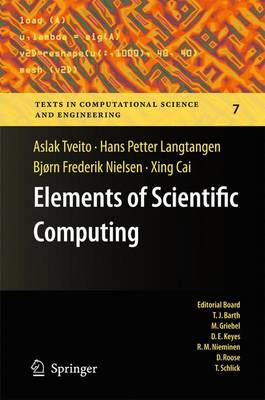•Elements of Scientific Computing - Texts in Computational Science and Engineering 7 (Hardback)

(author), (author), (author), (author)
£64.99
Hardback 468 Pages / Published: 27/09/2010
• We can order this

Usually dispatched within 3 weeks

Science used to be experiments and theory, now it is experiments, theory and computations. The computational approach to understanding nature and technology is currently flowering in many fields such as physics, geophysics, astrophysics, chemistry, biology, and most engineering disciplines. This book is a gentle introduction to such computational methods where the techniques are explained through examples. It is our goal to teach principles and ideas that carry over from field to field. You will learn basic methods and how to implement them. In order to gain the most from this text, you will need prior knowledge of calculus, basic linear algebra and elementary programming.

Publisher: Springer-Verlag Berlin and Heidelberg GmbH & Co. KG
ISBN: 9783642112980
Number of pages: 468
Weight: 881 g
Dimensions: 235 x 155 x 26 mm
Edition: 2010 ed.

MEDIA REVIEWS

From the reviews:

"This gentle introduction to scientific computing aims to convey the basic ideas, principles and techniques of computational science to undergraduates in mathematics, science and engineering. ... The real strength of the text is its adroit mix of analytical and numerical, theoretical and practical. ... This is a top-notch book on scientific computing written with clarity ... and a good sense of what students need to learn. It is among the best books in this area that I have seen." (William J. Satzer, The Mathematical Association of America, February, 2011)

"The text flows nicely, and Tveito (Simula Research Laboratory, Norway) and colleagues thoroughly explain different subjects in meaningful steps. In addition to exercises at the end of each chapter, an instructive `Projects' section allows readers to practice new concepts. ... Overall, this textbook is a welcome addition to a small set of books currently available in scientific computing, an expanding area for undergraduate course development in many US colleges. Summing Up: Recommended. Lower-division undergraduates through researchers/faculty." (D. Papamichail, Choice, Vol. 48 (9), May, 2011)

"This book is part of Springer's Texts in Computational Science and Engineering series, so it is written for students. ... chapters more or less serve as a limited introduction to computational mathematics: they cover basic algorithms in numerical integration, initial-value problems for scalar ODEs, initial-value problems for two-component ODEs, rootfinding in one or two dimensions, and least squares approximations using constants, lines, and parabolas."--- (Toby Driscoll, SIAM Review, Vol. 53 (4), 2011)

"In this appealing book the authors introduce to basic principles of numerical calculations which every engineer or scientist working with computers should be familiar with. ... a very good selection of topics treated and presented important methods in a gentle style ... ." (Rudolf Gorenflo, Zentralblatt MATH, Vol. 1220, 2011)